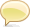# geo:region-affine-transform

```geo:region-affine-transform(
\$region as cts:region,
\$transform as map:map*,
[\$options as xs:string*]
) as cts:region```

### Summary

Perform an affine transformation on a geospatial region. The transformation is always applied in the raw coordinate system (Cartesian).

Parameters
\$region
\$transform A sequence of maps that specify the transformation to apply. Each map should contain at least one of the following transform specifications:
`translation`
Translate the points of the region by the given amount in the x and y coordinates. The value is a map with the following keys:
`tx`
Amount to shift the points in the x direction. (Required.)
`ty`
Amount to shift the points in the y direction. (Required.)
`scaling`
Scale the points of the region by the given amount of the x and y coordinates. The value is a map with the following keys:
`sx`
Amount to scale the points in the x direction. (Required.)
`sy`
Amount to scale the points in the y direction. (Required.)
`psc`
Point to scale relative to. (Optional. Default is the origin.)
`rotation`
Rotate the points of the region by the given angle. The value is a map with the following keys:
`angle`
Amount to rotate the points (radians). (Required.)
`prot`
Point to rotate relative to. (Optional. Default is the origin.)
`shearing`
Shear the points of the region by the given amounts. The value is a map with the following keys:
`shxy`
Amount of shearing due to x in y direction. (Required.)
`shyx`
Amount of shearing due to y in x direction. (Required.)
`reflection`
Reflect the points of the region around a line or a point. The value is a map with the following keys:
`lineR`
Line of reflection.
`pref`
Point to reflect points around. The value is a map with the following keys:
`start`
The starting point of the line.
`end`
The ending point of the line.
Exactly of `lineR` or `pref` must be specified.
If no transform specifications are provided, the points are transformed by the identity transform. That is, they remain unchanged.
\$options Geospatial options that affect the transformation. Currently there are no relevant options.

### Usage Notes

The ordering of transformations applied makes a difference. For example, scaling the point (1,1) by x=2 and y=3 and then translating it by x=1 and y=1 gives us the point (4,4). But translating it by x=1 and y=1 and then scaling it by x=2 and y=3 gives us (4,5). When multiple transformation are mentioned in a single map, the ordering is: translation, scaling, rotation, shearing, and then reflection. In general it is better to put separate transformations in separate maps in the sequence so there is no confusion. Transforms in the sequence are applied in order.

### Example

```(: Reflection about the line from point (2.5,0) to point (2.5,2.0) :)
geo:region-affine-transform(
cts:polygon((
cts:point(1.5,0),cts:point(2.5,1),cts:point(1.5,2),
cts:point(0.5,2),cts:point(0.5,0),cts:point(1.5,0))),
xdmp:from-json-string('
{reflection: { lineR: {start: [2.5,0], end:[2.5,2.0]} }
}'))

=> 3.5,0 2.5,1 3.5,2 4.5,2 4.5,0 3.5,0
```Stack Overflow: Get the most useful answers to questions from the MarkLogic community, or ask your own question.

## CommentsThe commenting feature on this page is enabled by a third party. Comments posted to this page are publicly visible.